# Shapes of Molecules (VSEPR theory) Chemistry Tutorial

## Key Concepts

• A molecule consists of 2 or more atoms joined together by covalent bonds.
• A covalent bond consists of 2 electrons shared between the 2 atoms (a bonding pair of electrons)
• The shape of a molecule, its geometry, is a description of the way the atoms in the molecule occupy space.
• A diatomic molecule, a molecule composed of only 2 atoms, must always be linear in shape as the centres of the 2 atoms will always be in a straight line.
• Valence Shell Electron Pair Repulsion (VSEPR) theory is used to predict shapes and bond angles of simple molecules.
• Valence Shell Electron Pair Repulsion (VSEPR) theory is also referred to as 'Electron Cloud' Repulsion Theory

No ads = no money for us = no free stuff for you!

## Valence Shell Electron Pair Repulsion (VSEPR) Theory

Valence Shell Electron Pair Repulsion theory (VSEPR theory, which is usually pronounced 'vesper'), also known as 'electron cloud' repulsion theory, is a method used to predict the shape of a molecule.

Valence shell electron pair repulsion theory rests on 3 assumptions:

1. Electron pairs in the valence shell of an atom orient themselves so that their total energy is minimized.

Electron pairs approach the nucleus of the atom as closely as possible while at the same time they stay away from each other as far as possible.
Remember that the pairs of dots in a Lewis structure (electron dot diagram) represent pairs of valence electrons (an 'electron cloud'). These electron pairs can be either:

• lone pairs (non-bonding pairs) of electrons
(those electron pairs not used in the formation of covalent bonds)
• bonding pairs of electrons
(those pairs of electrons shared between 2 atoms in the formation of a covalent bond)

2. Repulsion between 2 lone pairs of electrons is greater than the repulsion between a bonding pair and a lone pair of electrons, the repulsion between 2 bonding pairs is the weakest repulsion.

Remember that electrons carry a negative charge, therefore these pairs of electrons ('electron clouds') will repel each other electrostatically.
In order of decreasing electrostatic repulsion:

 strongest repulsion intermediate repulsion weakest repulsion lone pair - lone pair > lone pair - bonding pair > bonding pair - bonding pair

3. The smaller the angle between 2 adjacent electron pairs, the greater the electrostatic repulsion.

 strongest repulsion intermediate repulsion weakest repulsion small angle between 2 adjacent electron pairs > intermediate angle > large angle between 2 adjacent electron pairs 90° between electron pairs > 120° between electron pairs > 180°between electron pairs

Do you know this?

Play the game now!

## Using VSEPR to Predict Molecular Shapes (Molecular Geometries)

Molecular shape is also referred to as molecular geometry.

Steps to determine the shape of a molecule:

1. Draw the Lewis structure (electron dot diagram)

2. Determine the total number of electron pairs in the valence shell of the central atom

The total number of electron pairs in the valence shell of a central atom in a covalent molecule is referred to as the steric number:

number of bonding pairs + number of lone pairs = total number of electron pairs = steric number

If the central atom obeys the octet rule there will be 4 pairs of electrons, that is, 4 × 2 electrons = 8 electrons, and the steric number will be 4:

number of bonding pairs + number of lone pairs = 4

3. Determine the number of lone pairs and position these around the central atom so that:

• lone pair - lone pair repulsions are minimised
(lone pairs as far away from each other as possible, that is, larger bond angles)
• lone pair - bonding pair repulsions are minimised
(lone pairs and bonding pairs as far away from each other as possible, that is, any bonding pairs will be pushed closer together)

The table below summarizes some common molecular shapes given the central atom's steric number (that is, given the total number of pairs of electrons in the valence shell of the central atom)1:

Total Number of electron pairs
(Steric number)
Arrangement of electron pairs Number of bonding pairs of electrons Number of lone pairs of electrons Shape of Molecule
(Geometry of Molecule)
Name of Shape Bond Angle Examples
(1 or more) linear 1 (0 or more)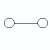linear 180o H2
HCl
2 linear 2 0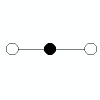linear 180o CO2
HCN
3 trigonal planar 3 0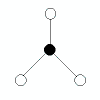triganol planar 120o BCl3
AlCl3
4 tetrahedral 4 0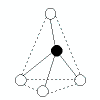tetrahedral 109.5o CH4
SiF4
3 1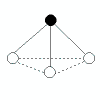trigonal pyramidal <109.5°
(bond angles in ammonia, NH3, are 107°)
NH3
PCl3
2 2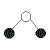bent <109.5°
(bond angles in water, H2O, are 105°)
H2O
SCl2
5 trigonal bipyramidal 5 0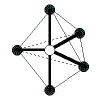trigonal bipyramidal 120o in the trigonal planar part of the molecule,
90o for the others
PCl5
6 octahedral 6 0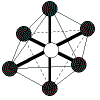octahedral 90o SF6

How to use the table:

1. Draw the Lewis structure (electron dot diagram) for your molecule and determine which atom is the central atom

2. Count the total number of number of electron pairs around the central atom (the steric number)
Identify the appropriate section of the table

For example: Steric number = 4
basis of arrangement of electrons is tetrahedral
(that is, the arrangement before repulsion between electron pairs is considered is tetrahedral)

3. Determine the number of bonding pairs
For example, for a steric number of 4 the possibilities are:

• 4 bonding pairs and 0 lone pairs - shape is tetrahedral
Justification: bonding pairs of electrons will repel each other so that they are as far away from each other as possible, ideally this corresponds to bond angles of 109.5° and the resulting shape is tetrahedral
• 3 bonding pairs and 1 lone pair - shape is trigonal pyramidal
Justification: greater lone pair - bonding pair repulsion increases bond angle between the lone pair and the bonding pairs to >109.5° therefore the bond angle between the bonding pairs decreases to less than 109.5° and the molecule is said to be trigonal pyramidal
• 2 bonding pairs and 2 lone pairs - shape is bent
Justification: greater repulsion between the 2 lone pairs of electrons increases the bond angle between them to >109.5° therefore the bond angle between the bonding pairs decreases to less than 109.5° and the molecule is said to be bent
• only 1 bonding pair of electrons and 3 lone pairs - shape is linear
Justification: if there are only 2 atoms in the molecule they must lie in the same plane

Do you understand this?

Take the test now!

## Worked Examples: Predict the Shape (geometry) of a Molecule Using VSEPR

What is the shape (geometry) of the hydrogen chloride, HCl, molecule?

1. Draw the Lewis structure (electron dot diagram) and identify the central atom
 H: ..Cl:.. Select the chlorine atom, Cl, as central atom
2. Determine the steric number (total number of electron pairs around the central chlorine atom)
steric number = 4
3. Determine the number of bonding pairs
1 bonding pair of electrons and 3 lone pairs of electrons
4. State the shape.
From the table the shape is linear: H-Cl
Justification: there are only 2 atoms in the molecule so the only way they can be arranged is by a bond between them which is a bond angle of 180°

What is the shape (geometry) of the hydrogen cyanide, HCN, molecule?

1. Draw the Lewis structure (electron dot diagram) and identify the central atom
 H :C ...... N: Carbon atom, C, is the central atom
2. Determine the steric number (total number of electron pairs around the central carbon atom)
steric number is 4
3. Determine the number of bonding pairs
4 bonding pairs of electrons around the central carbon atom, but, 3 of these pairs are between the carbon and nitrogen atom forming the triple covalent bond between the central carbon atom and the nitrogen atom, so we can treat this as 1 set of bonding pairs of electrons (or an electron cloud), which means there are 2 sets of bonding pairs of electrons (electron clouds) in total.
0 lone pairs of electrons around the central carbon atom
4. State the shape.
Shape is linear: H-C≡N:
Justification : 2 sets of bonding pairs of electrons will repel each other so that they get as far away from each other as possible, that is, the bond angle between them will be 180° and the molecule is linear

What is the shape (geometry) of the aluminium chloride, AlCl3, molecule?

1. Draw the Lewis structure (electron dot diagram) and identify the central atom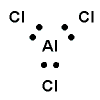Aluminium atom, Al, is the central atom.
2. Determine the steric number (total number of electron pairs around the central aluminium atom)
steric number is 3
3. Determine the number of bonding pairs
3 bonding pairs of electrons around the central Al atom.
0 lone pairs of electrons around the central Al atom.
4. State the shape.
Shape is trigonal planar :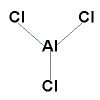Justification: there are no lone pairs of electrons around the central Al atom so the bonding pairs of electrons will repel each so that they will get as far away from each other as possible resulting in bond angles of 120° which results in a trigonal planar shape

What is the shape (geometry) of the methane, CH4, molecule?

1. Draw the Lewis structure (electron dot diagram) and identify the central atom
 H H ..:C:.. H Carbon atom, C, is the central atom H
2. Determine the steric number (total number of electron pairs around the central carbon atom)
steric number is 4
3. Determine the number of bonding pairs
4 bonding pairs of electrons around the central carbon atom.
0 lone pairs of electrons around the centration carbon atom.
4. State the shape.
Shape is tetrahedral
Justification: Since there are no lone pairs of electrons around the central carbon atom, all the bonding pairs will repel each other equally, getting away from each other as far as possible, resulting in bond angles of 109.5° and a tetrahedral shape.

What is the shape (geometry) of the ammonia, NH3, molecule?

1. Draw the Lewis structure (electron dot diagram) and identify the central atom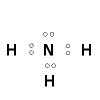Central atom is the nitrogen, N, atom.
2. Determine the steric number (total number of electron pairs around the central nitrogen atom)
steric number is 4
3. Determine the number of bonding pairs
3 bonding pairs of electrons around the central N atom
1 lone pair of electrons around the central N atom
4. State the shape.
Shape is trigonal pyramidal: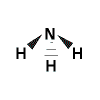In the representation above, the solid triangles represent bonds coming out of the plane of the screen, the broken lines represent a bond going behind the plane of the screen.

Justification: repulsion is greatest between the lone pair and the bonding pairs of electrons so the angle between these will be greater than 109.5° which means the remaining bonding pairs of electrons will be forced closer to each other resulting in bond angles less than 109.5° and a trigonal pyramidal shape.

What is the shape (geometry) of the water, H2O, molecule?

1. Draw the Lewis structure (electron dot diagram) and identify the central atom
 H ..:O:.. H Oxygen atom, O, is the central atom
2. Determine the steric number (total number of electron pairs around the central oxygen atom)
steric number is 4
3. Determine the number of bonding pairs
2 bonding pairs of electrons around the central O atom
2 lone pairs of electrons around the central O atom
4. State the shape.
Shape is bent: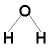In the representation above, solid lines represent bonds that are in the same plane as the screen.

Justification: Lone pair - lone pair repulsion is greater than lone pair - bonding pair repulsion so the two lone pairs of electrons will force each other to get as far away from each other as possible which results in a bond angle between the lone pairs of greater than 109.5° while the 2 bonding pairs of electrons are forced closer to each other resulting in a bond angle between them of less than 109.5°, and the final shape of the molecule is bent.

Can you apply this?

Join AUS-e-TUTE!

Take the exam now!

Footnotes

1. This discussion assumes that there are a maximum of 2 elements present in the atom. 1 element is the central atom of the structure, any atoms bonded to this central atom will be atoms of the same element. If there are more than 2 elements present in the molecule, distortions from the ideal geometry amy result.